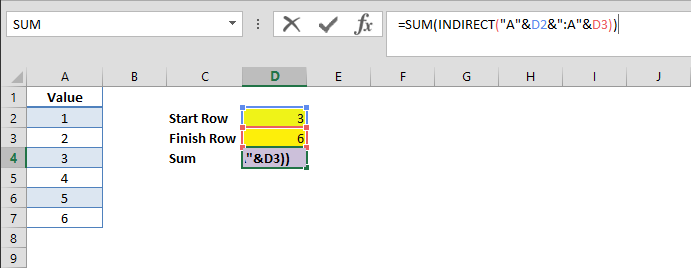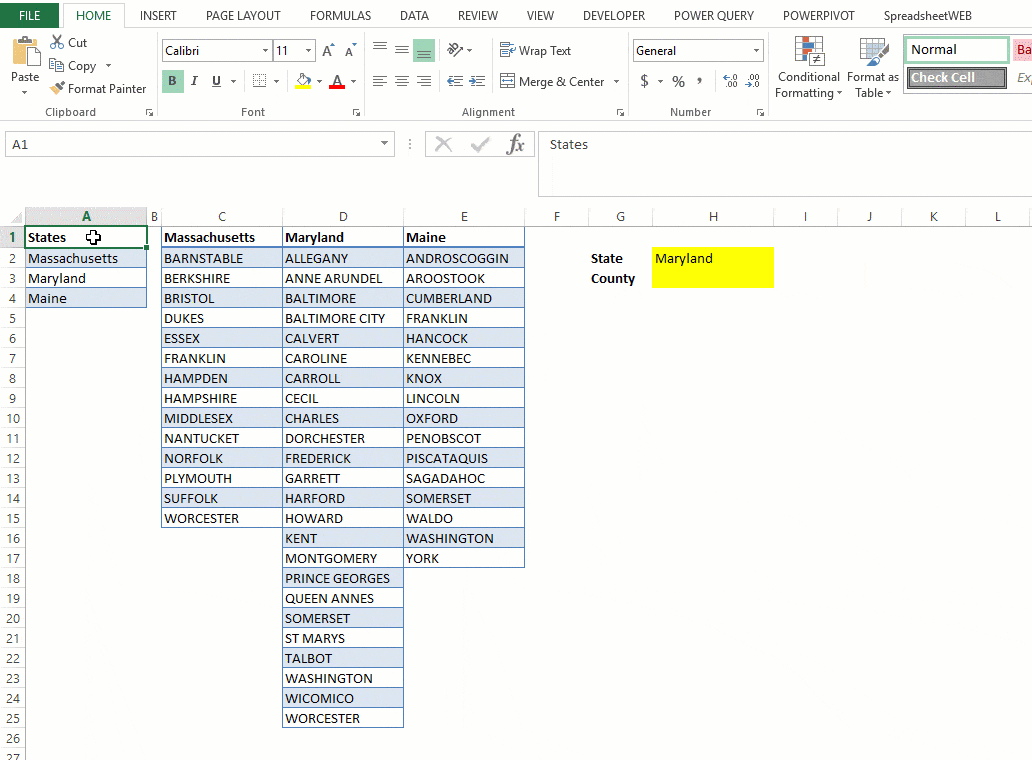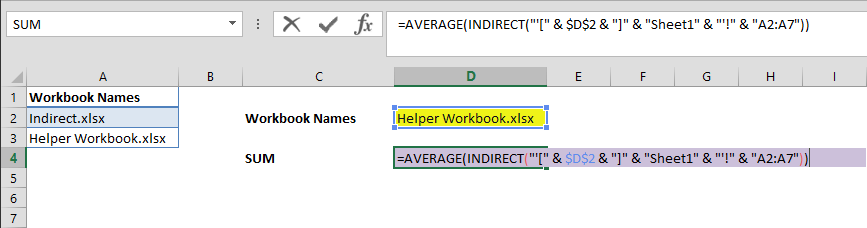INDIRECT is a versatile function in Excel’s already huge repertoire of referencing tools. In this guide we're going to take a look at its various use cases. This function can return the reference specified by a text string, foolproof your Excel models, or even pull data from other sources. This function has 2 arguments.Taking a look at the formula’s anatomy, we see that the first parameter is “ref_text”. This is the string that’s going to be used to create the range reference. The reference can be a cell, a range of cells, or a named range.

The second, and optional parameter is “[a1]”. This is a Boolean (TRUE/FALSE) argument that specifies whether ref_text is A1-style reference or R1C1-style reference. It’s TRUE (or “1”) by default and its default behavior is A1-style. These two styles represent two types of cell coordinate reference. We will cover this in detail later. Please feel free to download our sample spreadsheets from here and here.

#### Returning Single Cell Value

Let’s start with basic use cases of the INDIRECT formula. This handy formula can be used to return a reference by entering a ref_text value (a cell reference). For instance, entering “A2” (with the quotation marks) will simply print the value of the cell A2.Using a cell reference which contains a cell reference as string will return the cell value, because cell C3 includes the string “A2” (with the quotation marks). As a result, the function returns cell A2’s value.#### Cell Reference with a1 = TRUE

In the first use case, we demonstrated that the [a1] argument is optional. [a1] parameter is not required if ref_text is entered in A1-style. A1-style refers to the traditional cell coordinates in Excel (i.e. C4 refers to column C, row 4).#### Cell Reference with a1 = FALSE (R1C1 reference)

[a1] argument should be defined as FALSE or “0” if ref_text is entered in R1C1-style. R1C1-style refers to entering a cell coordinate with row and column numbers (i.e. R4C3 means fourth row, third column, or C3 in the A1-style). Entering “R2C1” into the first parameter of our INDIRECT formula will return the value of cell A2.#### Returning a Range

INDIRECT function can return an entire range. Being a volatile formula, it can even work with dynamic references. Let’s take an example.

=SUM(INDIRECT("A"&D2&":A"&D3))

formula sums the values from the selected range. This formula first calculates the string, "A"&D2&":A"&D3

This returns “A3:A6”. Then, it continues with the second part, where the formula becomes, =SUM(A3:A6)

The final result is “14”.

Because the formula we created is tied to the cells D2 and D3; the sum result can be easily manipulated by changing the referenced cells. For example; if we change D2 to 4, and D3 to 7, the new result will be 18.### Using INDIRECT with Named Ranges and Dynamic Data Validation

INDIRECT function can be used with named ranges as well as other functions. Let’s assume that we have a named range called “my_name”.

=INDIRECT(my_name)

formula will return the value, or reference produced by the named range “my_name”. This feature can be used to create dynamic Data Validation Lists (also known as combo boxes). Let’s create a combo box type of list that updates options based on the state selection.

We need to begin with copying the state and county names as separate lists. In this example, we’re going to use Massachusetts, Maryland, and Maine. The state list (A2:A4 in our example) will be the state selection combo box. County lists should be identified as named ranges individually. Named ranges should match the state names to be used. For instance,

1. Massachusetts C2:C15
2. Maryland D2:D25
3. Maine E2:E17

Next, we must create a Data Validation List (combo-box) for state selection. To do this, select the State input, and go to the Data ribbon on the top Excel menu. Here, click Data Validation and select “List”, for the Allow type menu. Then, select the state names (A2:A4 in our example) for the Source. Press OK when you’re done.In this next step, we’re going to create a “dynamic” Data Validation List (combo-box) for counties. To make a combo-box dynamic, follow the steps from the previous list, but use the INDIRECT function instead of selecting Source from the spreadsheet. Add

=INDIRECT(\$H\$2)

formula to the Source field. Formula will return the reference of cell H2, which contains named ranges of counties.Now, the county list in H3 will update to match our selection when we choose a state from the combo box (H2).### Dynamic Worksheet & Workbook References

Most Excel gurus keep their data in a separate worksheets to keep their business model organized, and create a clean workflow. INDIRECT formula conveniently allows you to access different worksheets and/or workbooks when building formulas. This can be done by using generated worksheet or workbook paths.

#### Worksheet

Worksheet name must be added with single quotes (‘’) and separate from the cell reference with an exclamation mark (!).

=INDIRECT("'" & \$D\$2 & "'!A2")

This formula gets cell A2 values from the worksheet which is selected in cell D2 by name. If cell D2’s value is “Cell”, this formula will be evaluated as

=INDIRECT("'Cell'!A2")### Workbook

Same method with worksheets can be used with workbooks. However, there are 2 additional rules to add workbook names into an address reference,

1. Workbook names must be used with their file extensions.
2. Workbook names must be separated with brackets (i.e. [x]).

=AVERAGE(INDIRECT("'[" & \$D\$2 & "]" & "Sheet1" & "'!" & "A2:A7"))

This function returns average value of numbers from the A2:A7 range of Sheet1 in the referenced workbook. If cell D2 is “Helper Workbook.xlsx” then the formula will become

=AVERAGE(INDIRECT("'[Helper Workbook.xlsx]Sheet1'!A2:A7"))#### Workbook “Helper Workbook.xlsx”#### Workbook “Indirect.xlsx”If your “ref_text” parameter is using references from another workbook (an external reference), make sure the other workbook is open on your machine. Otherwise, INDIRECT will give a #REF! error. You will get the same error if “ref_text” is referring to a cell range outside the row limit (1,048,576), or the column limit of 16,384 (XFD).

Although inexperienced users might find it hard to troubleshoot its issues, INDIRECT is a powerful formula Excel experts like utilizing at every opportunity. This nifty function will save you a lot of headache and give your spreadsheets advanced capabilities such as preventing user errors, or precision referencing techniques.